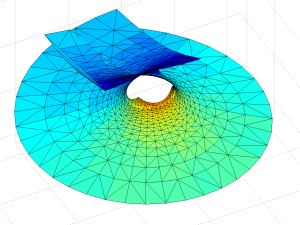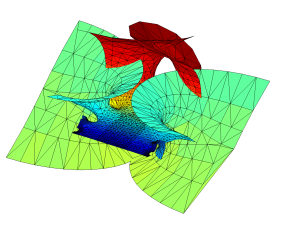# Håkan’s surface

Here is a minimal surface based on the Weierstrass-Enneper representation$f(z)=1, g(z)=\tanh^2(z)$. Written explicitly as a function from the complex number z to 3-space it is$\Re([-\tanh(z)(\mathrm{sech}^2(z)-4)/6,i(6z+\tanh(z)(\mathrm{sech}^2(z)-4))/6,z-\tanh(z)])$.

It is based on my old tanh surface, but has a wilder style. It gets helped by the fact that my triangulation in the picture is pretty jagged. On one hand it has two flat ends, but also a infinite number of catenoid openings (only two shown here).

I call it Håkan’s surface, since I came up with it on my dear husband’s birthday. Happy birthday, Håkan!

# A prime minimal surface

A short while ago I mentioned lacunary functions, complex functions that are analytic inside a disc but cannot be continued outside it. Then I remembered the wonderful Weierstrass-Enneper representation formula, which assigns a minimal surface to (nearly) any pair of complex functions. What happens when you make a minimal surface from a lacunary function?

I was not first with this idea. In fact, F.F. de Brito used this back in 1992 to demonstrate that there exist complete embedded minimal surfaces in 3-space that are contained between two planes.

Here is the surface defined by the function$g(z)=\sum_{p \mathrm{is prime}} z^p$, the Taylor series that only includes all prime powers, combined with$f(z)=1$.

Close to zero, the surface is flat. Away from zero it begins to wobble as increasingly high powers in the series begin to dominate. It behaves very much like a higher-degree Enneper surface, but with a wobble that is composed of smaller wobbles. It is cool to consider that this apparently irregular pattern corresponds to the apparently irregular pattern of all primes.

# Gamma function surfaces

The gamma function has a long and interesting history (check out (Davis 1963) excellent review), but one application does not seem to have shown up: minimal surfaces.

A minimal surface is one where the average curvature is always zero; it bends equally in two opposite directions. This is equivalent to having the (locally) minimal area given its boundary: such surfaces are commonly seen as soap films stretched from frames. There exists a rich theory for them, linking them to complex analysis through the Enneper-Weierstrass representation: if you have a meromorphic function g and an analytic function f such that$fg^2$ is holomorphic, then$X(z)=\Re\left(\int_{z_0}^z f(1-g^2)/2 dz\right)$$Y(z)=\Re\left(\int_{z_0}^z if(1+g^2)/2 dz\right)$$Z(z)=\Re\left(\int_{z_0}^z fg dz\right)$

produces a minimal surface$(X(z),Y(z),Z(z))$.

When plugging in the hyperbolic tangent as g and using f=1 I got a new and rather nifty surface a few years back. What about plugging in the gamma function? Let$f=1, g=\Gamma(z)$.

We integrate from the regular point$z_0=1$ to different points$z$ in the complex plane. Let us start with the simple case of$\Re(z)>1/2$.

The surface is a billowing strip, and as we include z with larger and larger real parts the amplitude of the oscillations grow rapidly, making it self-intersect. The behaviour is somewhat similar to the Catalan minimal surface, except that we only get one period. If we go to larger imaginary parts the surface approaches a horizontal plane. OK, the surface is a plane with some wild waves, right?

Not so fast, we have not looked at the mess for Re(z)<0. First, let’s examine the area around the z=0 singularity. Since the values of the integrand blows up close to it, they produce a surface expanding towards infinity – very similar to a catenoid. Indeed, catenoid ends tend to show up where there are poles. But this one doesn’t close exactly: for re(z)<0 there is some overshoot producing a self-intersecting plane-like strip.Gamma function minimal surface close to the z=0 singularity. Colour denotes Re(z). Integration contours from 1 to z run clockwise for Im(z)<0 and counterclockwise for Im(z)>0.

The problem is of course the singularity: when integrating in the complex plane we need to avoid them, and depending on the direction we go around them we can get a complex phase that gives us an entirely different value of the function. In this case the branch cut corresponds to the real line: integrating clockwise or counter-clockwise around z=0 to the same z gives different values. In fact, a clockwise turn adds [3.6268i, 3.6268, 6.2832i] (which looks like$\gamma\pi$ – a rather neat residue!) to the coordinates: a translation in the positive y-direction. If we extend the surface by going an extra turn clockwise or counterclockwise a number of times, we get copies that attach seamlessly.Gamma minimal surface patch that can be repeated by translation along the y-axis. Colour denotes Re(z).

OK, we have a surface with some planar strips that turn wobbly and self-intersecting in the x-direction, with elliptic catenoid ends repeating along the y-direction due to the z=0 singularity. Going down the negative x-direction things look plane between the catenoids… except of course for the catenoids due to all the other singularities for$z=-1,-2,\ldots$. They also introduce residues along the y-direction, but different ones from the z=0 – their extensions of the surface will be out of phase with each other, making the fully extended surface fantastically self-intersecting and confusing.

Of course, there may be related nifty surfaces.$1/\Gamma(z)$ is nicely behaved and looks very much like the Enneper surface near zero, with “wings” that oscillate ever more wildly as we move towards the negative reals. No doubt there are other beautiful things to look for in the vicinity.